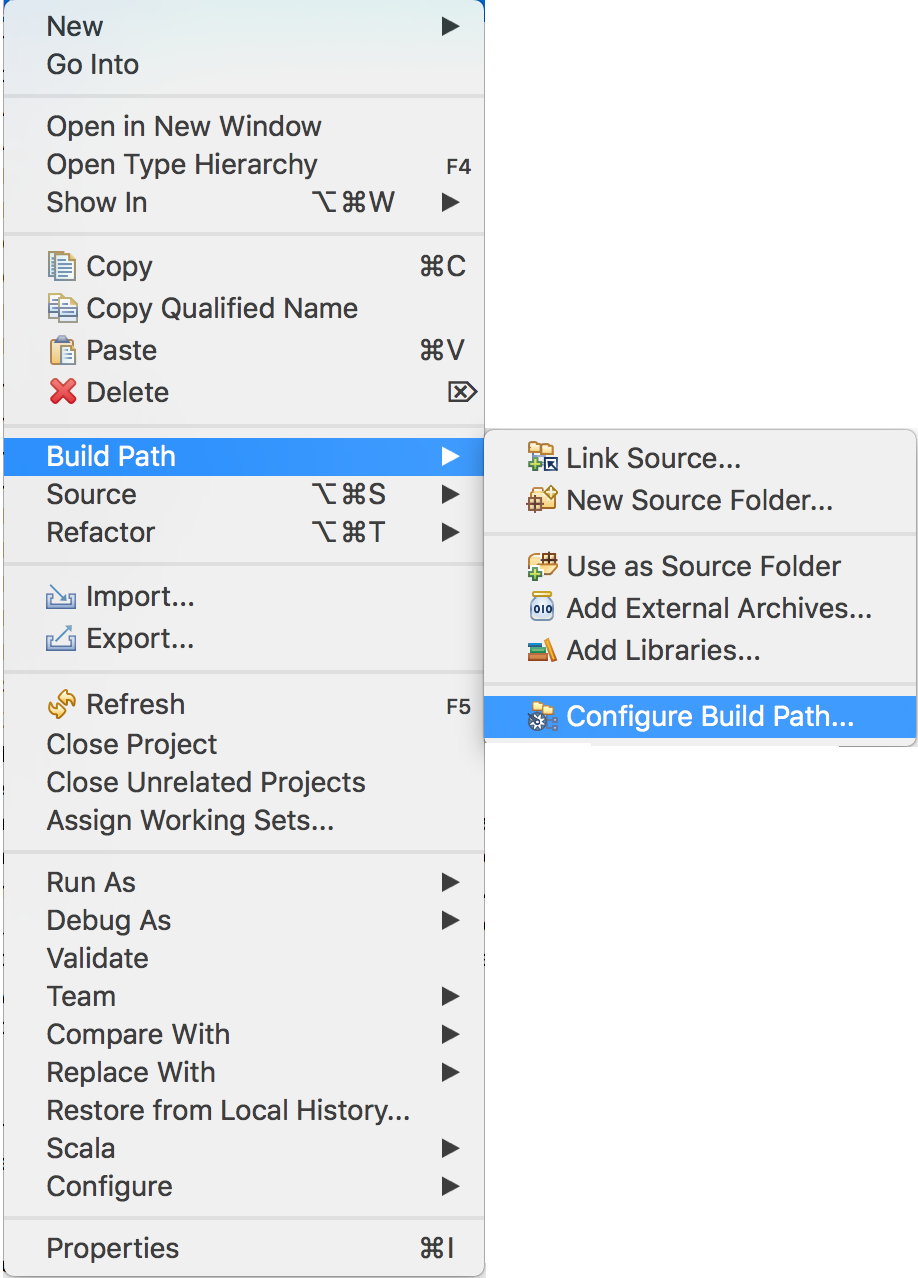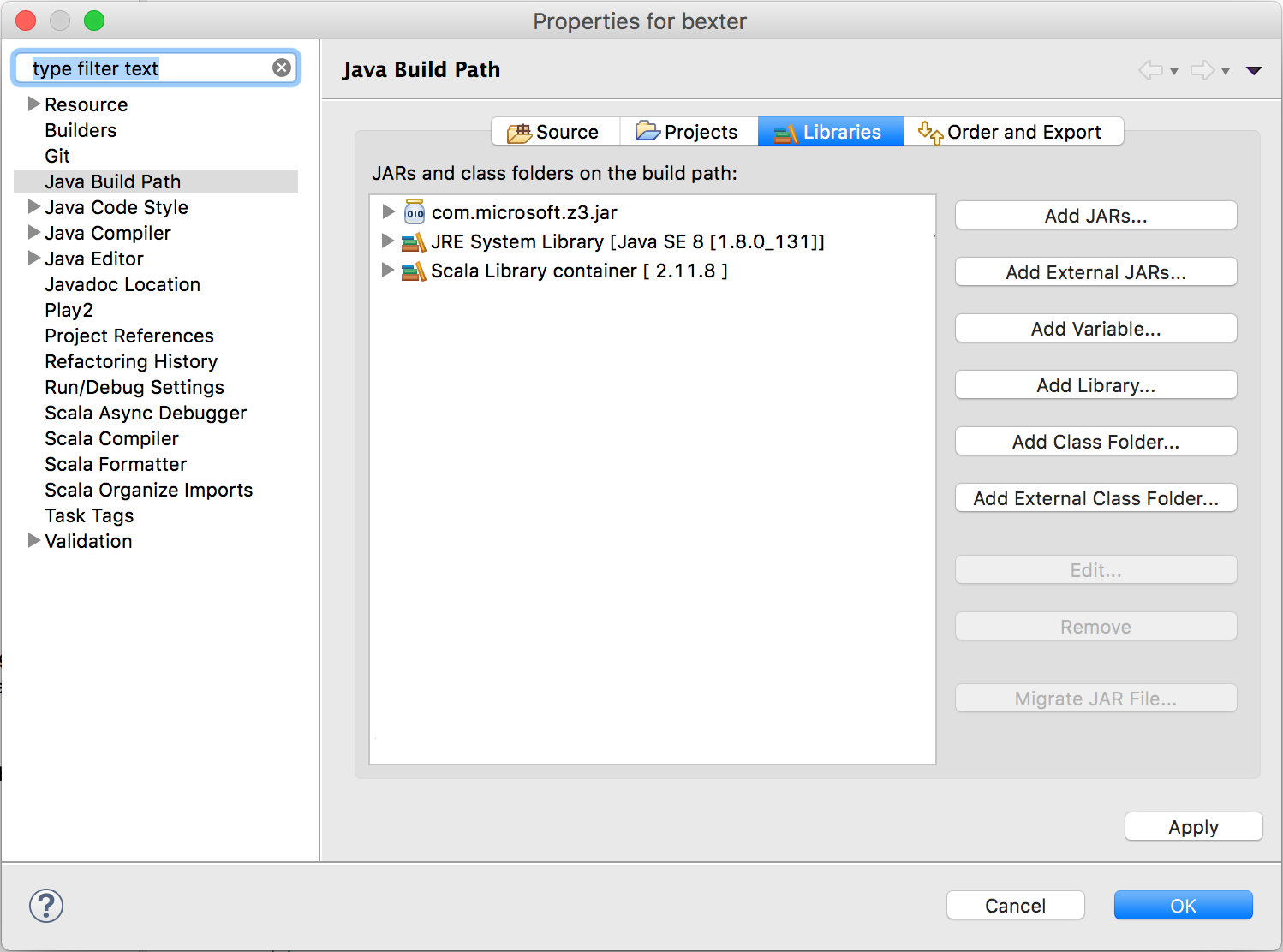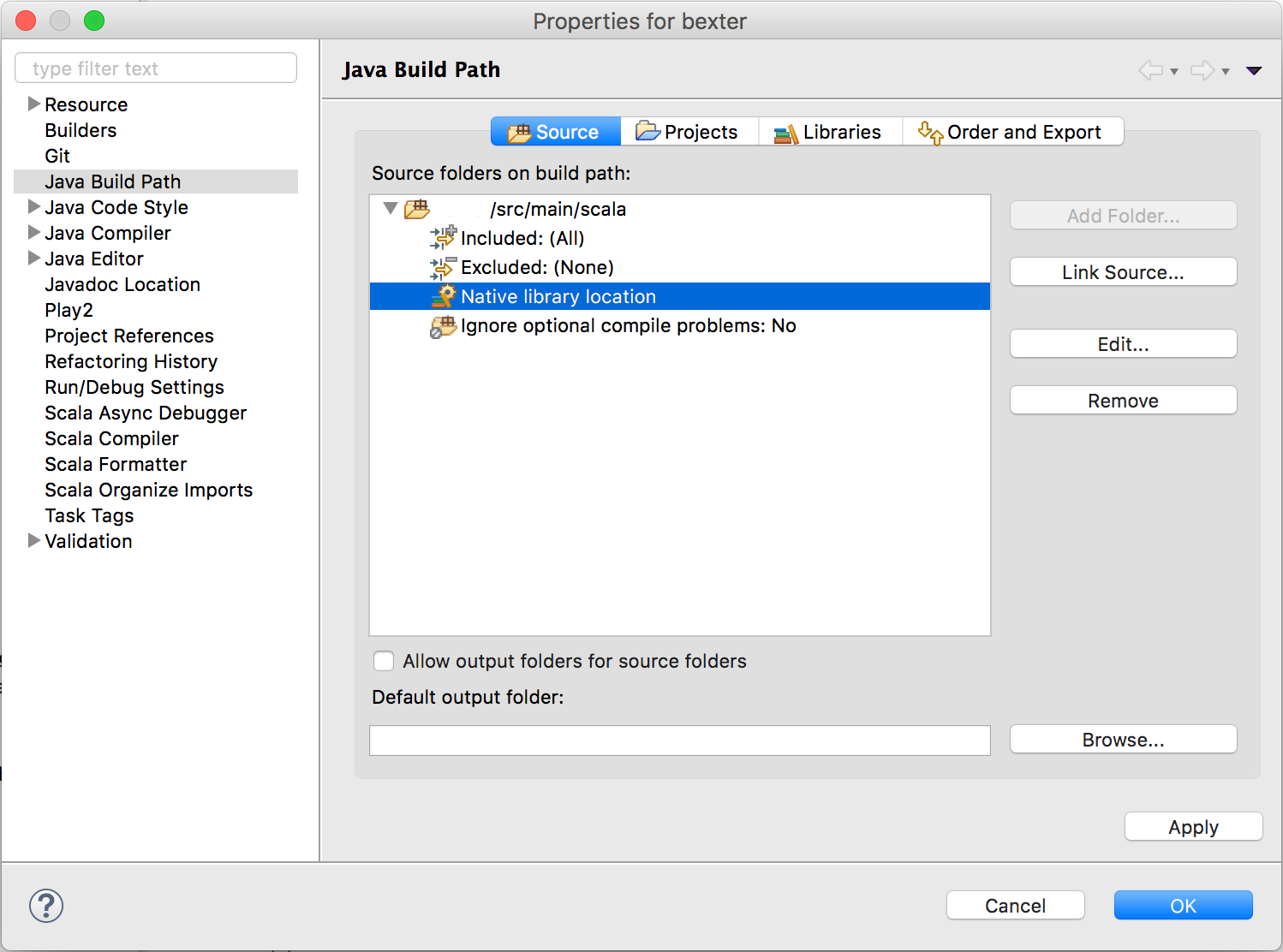# [JAVA] How to use Z3 library in Scala with Eclipse

Introduction In this post, I will show how to use the z3 Java library with Scala in Eclipse to solve a SAT formula. I made this post because even if it is quite short, I did lot of investigations on it to succeed to use z3 Java library with Scala in Eclipse IDE.

Requirements

• Python installed

Getting Z3 jar file

2. Go in the z3 folder and run the command `python scripts/mk_make.py --java`
3. Inside the z3 folder, go into the folder `build` and then `make examples`
1. Note: If you encounter an error during the `make` due to an invalid argument `-std=c++11`, edit the file `config.mk` and remove the argument `-std=c++11` from the `CXXFLAGS` flag
4. The jar file is now created in the folder `yourf3folder/build` and some Java examples

Note: For a full installation of z3 repository please follow these instructions.

Eclipse configuration In Eclipse, right-click on your Scala project, click on `Build Path` and `Configure Build Path`:It should open a window, once it's open go into the `Libraries` tab, click on `Add External JARs...` and select the z3 Jar file from the `gitfolder/build` (the file name would be `com.microsoft.z3.jar`):Once you did that, you have to specify one last thing to Eclipse to make it works with z3. On the same window as the previous configuration, go on the `Source` tab click on your project to open the menu context, choose `Native library location`, click on the button `Edit...` on the right and choose the `gitfolder/build` folder :You need to do that because the library uses two file into the `gitfolder/build` to work. (`libz3.dylib` and `libz3java.dylib` in Mac Os, `libz3.dll` and `libz3java.dll` in Windows)

Now you just have to import the library into your project file to use it. Because Scala is made with Java, you can also use this part of the tutorial to use the z3 library into a Java project in Eclipse. The below part of the tutorial was made for Scala but if you want to know how to do it in Java you can follow this link for Java examples.

How to use Z3 in Scala

There are two ways to use Z3 in Scala:

1. using a file SMT2 formatted with the formula you want to solve,
2. programming directly in code the formula.

In the following, I explain both ways by solving the propositional formula `((a | b) & c)`, as an example.

To solve a formula in z3 library, you need two things, the formula you want to solve in `BoolExpr` format and the variables from the formula you want to know the result format.

1. Using external SMT2-formatted files

Consider the following SMT2 formatted text file, named sample.smt2, which express the above propositional formula, i.e., `(a|b) & c)`:

#### `sample.smt2`

``````
(declare-const a Bool)
(declare-const b Bool)
(declare-const c Bool)
(assert (and (or a b) c))
``````

A Scala program code which read this smt2 file, and compute the formula is as follows:

``````
import com.microsoft.z3._

object z3test
{
def main(args: Array[String])
{
val ctx: Context = new Context(new java.util.HashMap[String, String])
val formula: BoolExpr = ctx.parseSMTLIB2File("sample.smt2", null, null, null, null)
val args: Array[Expr] = formula.getArgs // Here I will get 2 args
val arg1: Expr = args(0)
val arg2: Expr = args(1)

val a: Expr = formula.getArgs()(0).getArgs()(0)
val b: Expr = formula.getArgs()(0).getArgs()(1)
val c: Expr = formula.getArgs()(1)

/* Evaluation part */
val solver: com.microsoft.z3.Solver = ctx.mkSolver()   // Get a solver from the Context object

if(solver.check == Status.SATISFIABLE)                 // Check if the formula is satisfiable or not
{
val model: Model = solver.getModel                   // Get a model from the solver
val resultVa: Expr = model.eval(a,false)             // Get the result for each variables
val resultVb: Expr = model.eval(b,false)
val resultVc: Expr = model.eval(c,false)

println(resultVa)   // Result is true
println(resultVb)   // Result is false
println(resultVc)   // Result is true
}
}
}
``````

The program code first initializes a `Context` object with a `HashMap[String, String]` :

``````val ctx: Context = new Context(new java.util.HashMap[String, String])
``````

So you can parse the file by calling this method on the Context object previously created :

``````val formula: BoolExpr = ctx.parseSMTLIB2File("smt2file.txt", null, null, null, null)
``````

After you did that you get my BoolExpr variable which contains the formula, you can access to the formula by this way :

``````val args: Array[Expr] = formula.getArgs // Here I will get 2 args
val arg1: Expr = args(0)
val arg2: Expr = args(1)

println(arg1)
println(arg2)

// Result
(or a b)
c
``````

With my formula, I will use this code to get my variables :

``````val a: Expr = formula.getArgs()(0).getArgs()(0)
val b: Expr = formula.getArgs()(0).getArgs()(1)
val c: Expr = formula.getArgs()(1)
``````

There is a better way to achieve that : The formula is divide part by part and you can access it by using the method `getArgs()`. This method will return you an `Array[Expr]`. You can call it again on the `Expr` you got from the array. Until there is a single element in the `Expr` the size of the array return by `getArgs()` will be greater than `0`. I.E. : if you want to get all the variables of the formula, you can achieve this using a method like that :

``````  def getAllVariable(a: Array[Expr]): List[Expr] =
{
if (a == null || a.size == 0)
Nil
else
}
``````
1. Programming the formula

Here is the Scala code of the second approach:

``````import com.microsoft.z3._

object z3test
{
def main(args: Array[String])
{
val ctx: Context = new Context(new java.util.HashMap[String, String])

val a: BoolExpr = ctx.mkBoolConst("a")
val b: BoolExpr = ctx.mkBoolConst("b")
val c: BoolExpr = ctx.mkBoolConst("c")

val formula: BoolExpr = ctx.mkAnd(ctx.mkOr(a,b),c)

/* Evaluation part */
val solver: com.microsoft.z3.Solver = ctx.mkSolver()   // Get a solver from the Context object

if(solver.check == Status.SATISFIABLE)                 // Check if the formula is satisfiable or not
{
val model: Model = solver.getModel                   // Get a model from the solver
val resultVa: Expr = model.eval(a,false)             // Get the result for each variables
val resultVb: Expr = model.eval(b,false)
val resultVc: Expr = model.eval(c,false)

println(resultVa)   // Result is true
println(resultVb)   // Result is false
println(resultVc)   // Result is true
}
}
}
``````

As you can see the code, the programming-the-formula ways share many things in common with the first approach, however, there are some differences. In the previous part, you get the formula first, in this part it will be the contrary: you get the variables first and the formula second.

The program code first initializes a `Context` object with a `HashMap[String, String]` :

``````val ctx: Context = new Context(new java.util.HashMap[String, String])
``````

To create variables, you have to call the method `mkBoolConst()` on the Context object, like that :

``````val a: BoolExpr = ctx.mkBoolConst("a")
val b: BoolExpr = ctx.mkBoolConst("b")
val c: BoolExpr = ctx.mkBoolConst("c")
``````

To program the formula, you need to use the variables and the boolean methods provide by the `Context` object:

``````val formula: BoolExpr = ctx.mkAnd(ctx.mkOr(a,b),c)
``````

Notice that there is a lot of boolean methods provides by the Context object like `ctx.mkImplies()` or `ctx.mkNot()`.

Sources Z3 Github Eclipse for Scala, Scala IDE